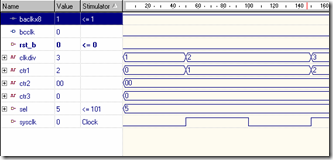Simulation of Arithmetic and Logic Unit (ALU)

Requirements:

• WARP
• Active HDL

Procedure:

• The Specifications of the Baud Rate are chosen.
• The input and the output ports of the above specification are defined to a Standard language (std_logic). The temporary variables are selected if necessary.
• Entity and Architecture is created for the above specification.
• The Result is verified by simulation and the waveforms are seen.

Program for Baud Rate Generator
Library IEEE;
Use IEEE.std_logic_1164.all;
Use IEEE.std_logic_unsigned.all;
Use IEEE.std_logic_arith.all;
Entity baud_gen_latest is
Port (
Sysclk: in STD_LOGIC;
rst_b: in STD_LOGIC;
sel: in STD_LOGIC_VECTOR (2 downto 0);
baclkx8: buffer STD_LOGIC;
bcclk: out STD_LOGIC
);
end baud_gen_latest;
architecture baud_gen_latest of baud_gen_latest is
Signal ctr1:std_logic_vector (3 downto 0):= "0000";
Signal ctr2:std_logic_vector (7 downto 0):= "00000000";
Signal ctr3:std_logic_vector (2 downto 0):= "000";
signal clkdiv:std_logic_vector(3 downto 0) := "0000";
begin
process(sysclk)
begin
if(sysclk'event and sysclk = '1') then
if(ctr1="1100") then ctr1 <= "0000";
else ctr1 <= ctr1 +1;
end if;
end if;
end process;
clkdiv <= ctr1 + 1;
process(clkdiv(3))
begin
if(rising_edge(clkdiv(3))) then
ctr2 <= ctr2 +1;
end if;
end process;
process(clkdiv(3))
begin
if (sel = "000") then
baclkx8 <= ctr2(0);
elsif sel = "001" then
baclkx8 <= ctr2(1);
end if;
end process;
process(baclkx8)
begin
if(rising_edge(baclkx8)) then
ctr3 <= ctr3 +1;
end if;
end process;
bcclk <= ctr3(2);
end baud_gen_latest;